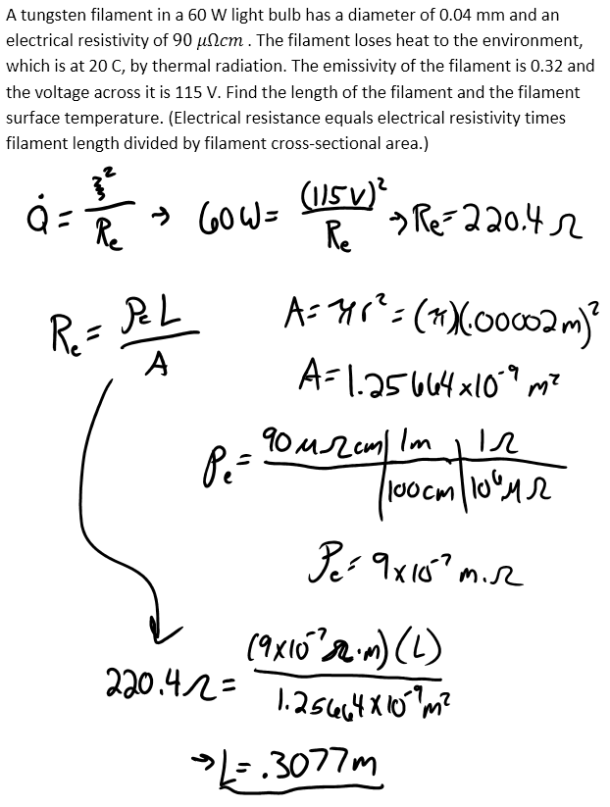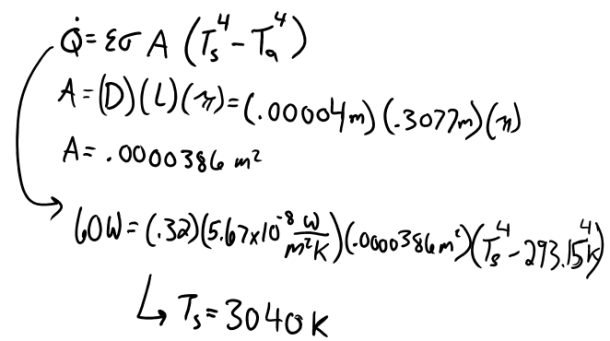heat and mass transfer problems engineering equations heat and mass transfer school homework engineering heat and mass transfer formulas heat and mass transfer solutions to heat and mass transfer problems full solution engineering problem solution heat and mass transfer math problems engineering equations heat and mass transfer school homework engineering solutions to heat and mass transfer formulas heat problems mass problem solutions to transfer problems full solution heat and mass transfer
heat and mass transfer problems engineering equations heat and mass transfer school homework engineering heat and mass transfer formulas heat and mass transfer solutions to heat and mass transfer problems full solution engineering problem solution heat and mass transfer math problems engineering equations heat and mass transfer school homework engineering solutions to heat and mass transfer formulas heat problems mass problem solutions to transfer problems full solution heat and mass transfer
Highalphabet Home Page heat and mass transfer problem solutions Heat and Mass Transfer Page
A tungsten filament in a 60 W light bulb has a diameter of 0.04 mm and an electrical resistivity of 90 micro ohm cm. The filament loses heat to the environment, which is at 20 C, by thermal radiation. The emissivity of the filament is 0.32 and the voltage across it is 115 V. Find the length of the filament and the filament surface temperature. (Electrical resistance equals electrical resistivity times filament length divided by filament cross-sectional area.)A tungsten filament in a 60 W light bulb has a diameter of 0.04 mm and an electrical resistivity of 90 micro ohm cm. The filament loses heat to the environment, which is at 20 C, by thermal radiation. The emissivity of the filament is 0.32 and the voltage across it is 115 V. Find the length of the filament and the filament surface temperature. (Electrical resistance equals electrical resistivity times filament length divided by filament cross-sectional area.)